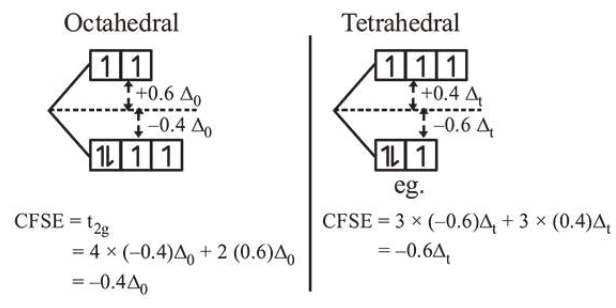# Solve the followingQuestion:

Consider that a $d^{6}$ metal ion $\left(\mathrm{M}^{2+}\right)$ forms a complex with aqua ligands, and the spin only magnetic moment of the complex is $4.90 \mathrm{BM}$. The geometry and the crystal field stabilization energy of the complex is :

1. octahedral and $-2.4 \Delta_{0}+2 \mathrm{P}$

2. tetrahedral and $-0.6 \Delta_{\mathrm{t}}$

3. octahedral and $-1.6 \Delta_{0}$

4. tetrahedral and $-1.6 \Delta_{t}+1 \mathrm{P}$

Correct Option: , 2

Solution:

Spin only magnetic moment $=4 \cdot 9=\sqrt{n(n+2)}$

$\therefore$ number of unpaired electrons $=4$

Two possible arrangements areSo, option (b) is correct.